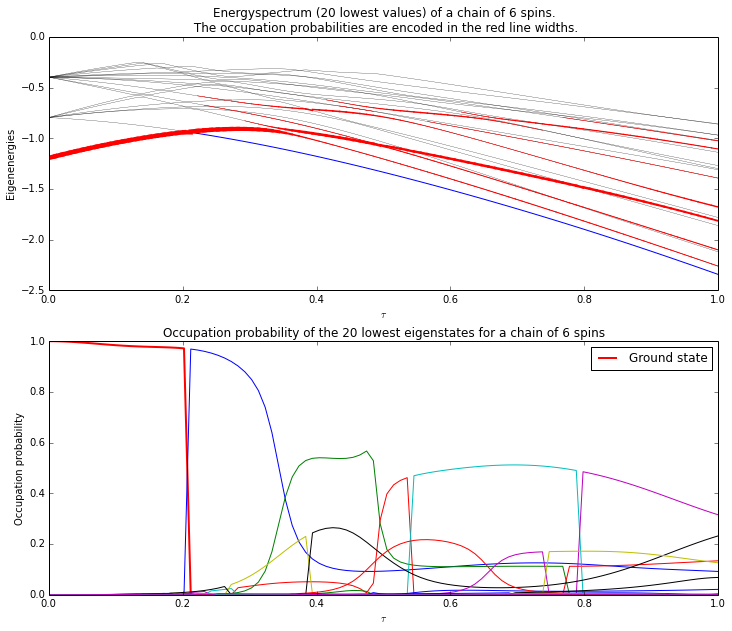# Lecture 8 - Adiabatic sweep¶

Author: J. R. Johansson ([email protected]), http://dml.riken.jp/~rob/

The latest version of this IPython notebook lecture is available at http://github.com/jrjohansson/qutip-lectures.

The other notebooks in this lecture series are indexed at http://jrjohansson.github.com.

## Introduction¶

In adiabatic quantum computing, an easy to prepare ground state of a Hamiltonian $H_0$ is prepared, and then the Hamiltonian is gradually transformed into $H_1$, which is constructed in such a way that the groundstate of $H_1$ encodes the solution to a difficult problem. The transformation of $H_0$ to $H_1$ can for example be written on the form

$\displaystyle H(t) = \lambda(t) H_0 + (1 - \lambda(t)) H_1$

where $\lambda(t)$ is a function that goes from goes from $0$ to $1$ when $t$ goes from $0$ to $t_{\rm final}$.

If this gradual tranformation is slow enough (satisfying the adiabicity critera), the evolution of the system will remain in its ground state.

If the Hamiltonian is transformed from $H_0$ to $H_1$ too quickly, the system will get excited from the ground state the adiabatic computing algorithm fails.

In this notebook we explore the dynamics of a spin Hamiltonian that is transformed from a simple Hamiltonian with an easy to prepare ground state, into a random spin Hamiltonian with a complicated ground state.

In :
%matplotlib inline
import matplotlib.pyplot as plt
import numpy as np

In :
from qutip import *
from scipy import *


### Parameters¶

In :
N = 6   # number of spins
M = 20  # number of eigenenergies to plot

# array of spin energy splittings and coupling strengths (random values).
h  = 1.0 * 2 * pi * (1 - 2 * rand(N))
Jz = 1.0 * 2 * pi * (1 - 2 * rand(N))
Jx = 1.0 * 2 * pi * (1 - 2 * rand(N))
Jy = 1.0 * 2 * pi * (1 - 2 * rand(N))

# increase taumax to get make the sweep more adiabatic
taumax = 5.0
taulist = np.linspace(0, taumax, 100)


### Precalculate operators¶

In :
# pre-allocate operators
si = qeye(2)
sx = sigmax()
sy = sigmay()
sz = sigmaz()

sx_list = []
sy_list = []
sz_list = []

for n in range(N):
op_list = []
for m in range(N):
op_list.append(si)

op_list[n] = sx
sx_list.append(tensor(op_list))

op_list[n] = sy
sy_list.append(tensor(op_list))

op_list[n] = sz
sz_list.append(tensor(op_list))


### Construct the initial state¶

In :
psi_list = [basis(2,0) for n in range(N)]
psi0 = tensor(psi_list)
H0 = 0
for n in range(N):
H0 += - 0.5 * 2.5 * sz_list[n]


### Construct the Hamiltonian¶

In :
# energy splitting terms
H1 = 0
for n in range(N):
H1 += - 0.5 * h[n] * sz_list[n]

H1 = 0
for n in range(N-1):
# interaction terms
H1 += - 0.5 * Jx[n] * sx_list[n] * sx_list[n+1]
H1 += - 0.5 * Jy[n] * sy_list[n] * sy_list[n+1]
H1 += - 0.5 * Jz[n] * sz_list[n] * sz_list[n+1]

# the time-dependent hamiltonian in list-function format
args = {'t_max': max(taulist)}
h_t = [[H0, lambda t, args : (args['t_max']-t)/args['t_max']],
[H1, lambda t, args : t/args['t_max']]]


### Evolve the system in time¶

In :
#
# callback function for each time-step
#
evals_mat = np.zeros((len(taulist),M))
P_mat = np.zeros((len(taulist),M))

idx = 
def process_rho(tau, psi):

# evaluate the Hamiltonian with gradually switched on interaction
H = qobj_list_evaluate(h_t, tau, args)

# find the M lowest eigenvalues of the system
evals, ekets = H.eigenstates(eigvals=M)

evals_mat[idx,:] = real(evals)

# find the overlap between the eigenstates and psi
for n, eket in enumerate(ekets):
P_mat[idx,n] = abs((eket.dag().data * psi.data)[0,0])**2

idx += 1

In :
# Evolve the system, request the solver to call process_rho at each time step.

mesolve(h_t, psi0, taulist, [], process_rho, args)

Out:
Odedata object with sesolve data.
---------------------------------
states = True, expect = True
num_expect = 0, num_collapse = 0

## Visualize the results¶

Plot the energy levels and the corresponding occupation probabilities (encoded as the width of each line in the energy-level diagram).

In :
#rc('font', family='serif')
#rc('font', size='10')

fig, axes = plt.subplots(2, 1, figsize=(12,10))

#
# plot the energy eigenvalues
#

# first draw thin lines outlining the energy spectrum
for n in range(len(evals_mat[0,:])):
ls,lw = ('b',1) if n == 0 else ('k', 0.25)
axes.plot(taulist/max(taulist), evals_mat[:,n] / (2*pi), ls, lw=lw)

# second, draw line that encode the occupation probability of each state in
# its linewidth. thicker line => high occupation probability.
for idx in range(len(taulist)-1):
for n in range(len(P_mat[0,:])):
lw = 0.5 + 4*P_mat[idx,n]
if lw > 0.55:
axes.plot(array([taulist[idx], taulist[idx+1]])/taumax,
array([evals_mat[idx,n], evals_mat[idx+1,n]])/(2*pi),
'r', linewidth=lw)

axes.set_xlabel(r'$\tau$')
axes.set_ylabel('Eigenenergies')
axes.set_title("Energyspectrum (%d lowest values) of a chain of %d spins.\n " % (M,N)
+ "The occupation probabilities are encoded in the red line widths.")

#
# plot the occupation probabilities for the few lowest eigenstates
#
for n in range(len(P_mat[0,:])):
if n == 0:
axes.plot(taulist/max(taulist), 0 + P_mat[:,n], 'r', linewidth=2)
else:
axes.plot(taulist/max(taulist), 0 + P_mat[:,n])

axes.set_xlabel(r'$\tau$')
axes.set_ylabel('Occupation probability')
axes.set_title("Occupation probability of the %d lowest " % M +
"eigenstates for a chain of %d spins" % N)
axes.legend(("Ground state",));### Software versions:¶

In :
from qutip.ipynbtools import version_table

version_table()

Out:
SoftwareVersion
Python3.4.1 (default, Jun 9 2014, 17:34:49) [GCC 4.8.3]
matplotlib1.3.1
Cython0.20.1post0
IPython2.0.0
Numpy1.8.1
SciPy0.13.3
QuTiP3.0.0.dev-5a88aa8
OSposix [linux]
Thu Jun 26 15:12:57 2014 JST